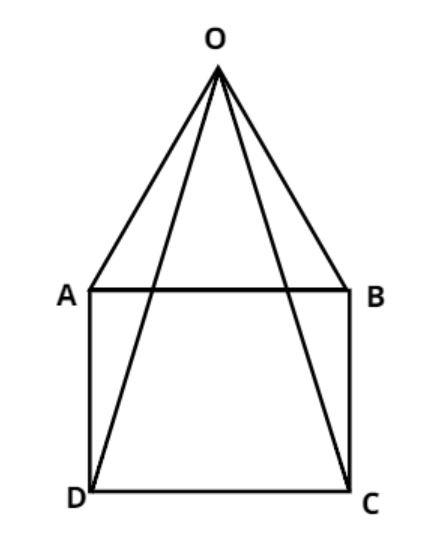Courses
Courses for Kids
Free study material
Free LIVE classes
MoreLIVE
Join Vedantu’s FREE Mastercalss

# A pyramid has a square base. How many edges does the pyramid have?$A.{\text{ 8}} \\ B.{\text{ 6}} \\ C.{\text{ 12}} \\ D.{\text{ 5}} \\$Verified
361.8k+ views
Hint: In this question first draw the pictorial representation it will give us a clear picture of what we have to find out and we all know that the square has four edges.The pictorial representation of the pyramid having a square base is shown.
And we all know that the square has four edges having four vertices.
Let us suppose ABCD is a square as shown in figure. So it has four edges having four vertices.
Now we have to construct the pyramid so for this all the vertices of the square meet at common point O as shown in figure.
So we can see after doing this there are four more edges which are OA, OB, OC and OD.
So, a pyramid having a square base has (4 + 4) = 8 edges.
So, this is the required answer.
Hence option (A) is correct.

Note: In such types of questions first draw the pictorial representation of the given problem then the number of edges having square base pyramid is the addition of edges of square and the edges when we meet all the vertices of the square to a common point O as above so the total no of edges having square base are 8 which is the required answer.
Last updated date: 30th Sep 2023
Total views: 361.8k
Views today: 5.61k